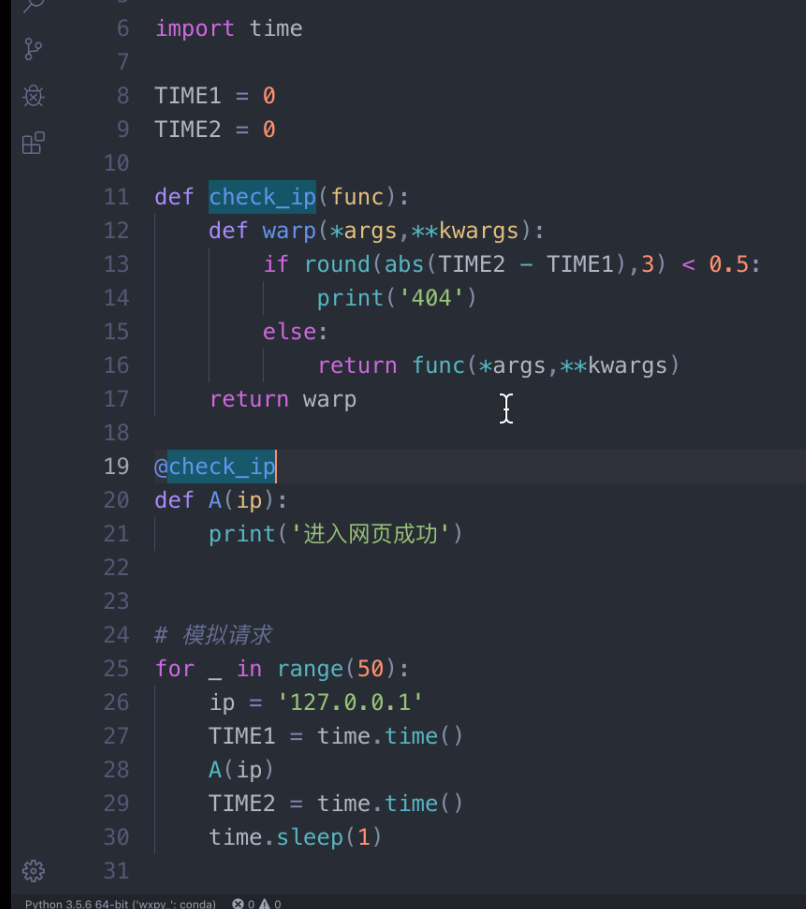# 学习python的第五天

## 一、知识点

1.PEP8编码规范，以及开发的一些建议
（1）代码编排

（2）import

• 不要使用 form xxx import *

• 标准库

• 第三方库

• 自定义库

• 单行不要多个库

• 模块用不到的不要去import

（3）空格

• ： ， ； 后面跟一个空格，前面无空格（行位分号后无空格）

（4）注释

• 忌：逐行添加注释，没有一个注释
• 行内注释：单行逻辑过于复杂时添加

2.* 和 **的用法

• 函数定义：
def fun(*args,**kwarg):
pass

• 参数传递：
def fun(x,y,z,a,b)：
print(x)
print(y)
print(z)
print(a)
print(b)
lst = [1, 2, 3]
dic = {'a': 11, 'b': 22)
fun(*lst, **dic)



3.python的赋值和应用

• ==， is : ==的判断是值，is判断的是内存地址（及对象的id）
• 小正数的对象：[-5, 256]
• copy, deepcopy的区别：
copy:只拷贝表层元素
deepcopy：在内存中重新创建所有子元素
4.迭代器，生成器
（1）迭代器、生成器的好处？
• 节省内存
• 惰性求值
（2）itertools
• 无限迭代
• 有限迭代
• 排列组合
5.装饰器
• 最简装饰器
def deco(func):
def warp(*args, **kwargs):
return func(*args, **kwargs)
return warp
@deco
def foo(a, b):
return a ** b


6.函数闭包

def fun():
l = []
def bar(i):
l.append(i)
return l
return bar
f1 = fun()
f2 = fun()



7.进程与线程
（1）进程：

（2）线程：

import multiprocessing

def A():
print(‘hello’)
if __name__ == "__main__":
#创建进程
p=multiprocessing.Process(target=A,args=())
p.star()  #启动进程
p.join()
print('Over')



（1）装饰器代码：

def deco(func):
pass
def warp(n,m):
sum=n+m
return func(sum,m)

return warp

@deco
def A(name,name2):
print(name)

A(10,20)



（2）

import random
def deco(func):
pass
def warp(n):
n=-1
print('验证码错误')
return func(n1,n2)

return warp

@deco
def A(n):
if n1==n2:
print('验证码正确')
else：
print('验证码错误')

num=random.range(1000,9999)
print('验证码为：%d'%num)
num2=int(input('>>'))
A(num,num2)


（3）流氓验证码：

import random
def deco2(parm):
def deco(func):
def warp(*args,**kwargs):
if parm:
kwargs['n1'] = -1
return func(*args,**kwargs)
return warp
return deco

@deco2(True)
def A(n1,n2):
if n1 == n2:
print('验证码正确')
else:
print('验证码错误')

num = random.randrange(1000,9999)
print('验证码是：%d'%num)
num2 = int(input('>>'))
A(n1=num,n2=num2)

#结果：

>>2222



（4）08-0553
12-061712-243280
06-183052
09-06199
11-30点击重新获取扫码支付余额充值